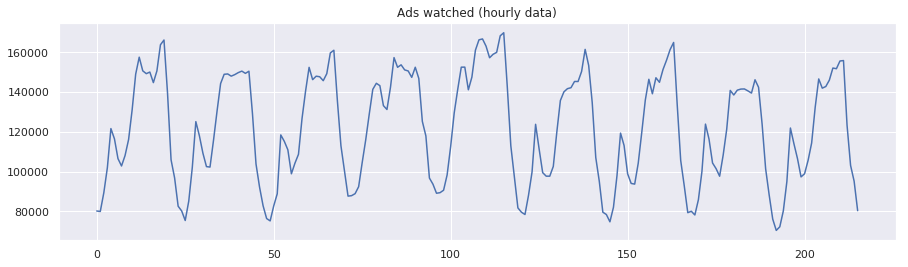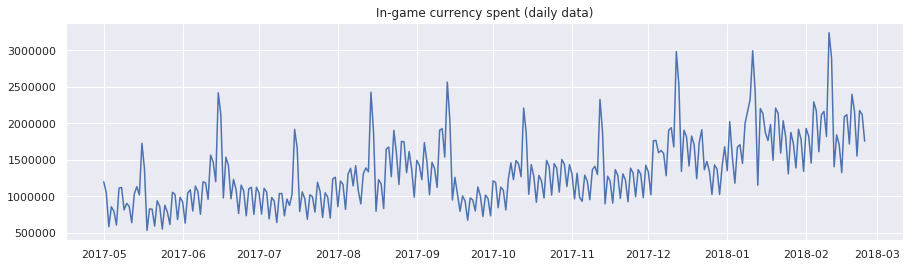## 时间序列处理与应用¶

• 时间序列
• SARIMA
• 线性回归
• XGBoost
• Prophet

### 时间序列数据介绍¶

In :
import warnings
from itertools import product
from dateutil.relativedelta import relativedelta
from tqdm import tqdm_notebook
from scipy import stats
import scipy.stats as scs
import statsmodels.api as sm
import statsmodels.tsa.api as smt
import statsmodels.formula.api as smf  # 统计与计量
from scipy.optimize import minimize  # 优化函数
import numpy as np
import pandas as pd
import matplotlib.pyplot as plt
import seaborn as sns
sns.set()
%matplotlib inline
warnings.filterwarnings('ignore')


In :
ads = pd.read_csv('../../data/ads.csv',
index_col=['Time'], parse_dates=['Time'])
index_col=['Time'], parse_dates=['Time'])

Out:
((216, 1), (300, 1))

In :
plt.figure(figsize=(15, 4))
plt.grid(True)
plt.show()In :
plt.figure(figsize=(15, 4))
plt.plot(currency.GEMS_GEMS_SPENT)
plt.title('In-game currency spent (daily data)')
plt.grid(True)
plt.show()